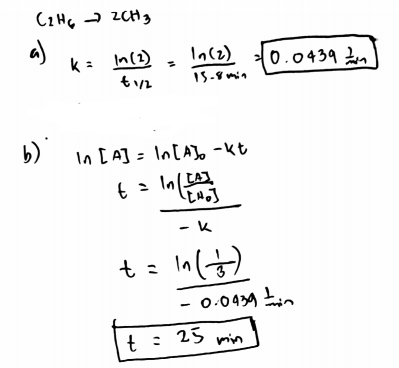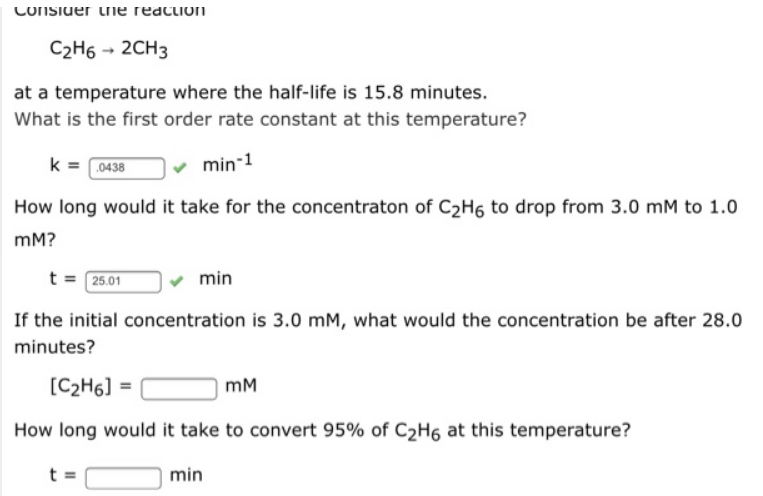# Problem: Consider the reactionC2H6 →2CH3at a temperature where the half-life is 15.8 minutes.What is the first order rate constant at this temperature?K = _____ min-1 How long would it take for the concentration of C2H6 to drop from 3.0 mM to 1.0 mN?T = _____ minIf the initial concentration is 3.0 mM? what would the concentration be after 28.0 minutes?[C2H6] = _____ mMHow long would it take to convert 95% of C2H6 at this temperature?t = _____ min

###### FREE Expert Solution92% (480 ratings)###### Problem Details

Consider the reaction

C2H6 →2CH3

at a temperature where the half-life is 15.8 minutes.

What is the first order rate constant at this temperature?

K = _____ min-1

How long would it take for the concentration of C2H6 to drop from 3.0 mM to 1.0 mN?

T = _____ min

If the initial concentration is 3.0 mM? what would the concentration be after 28.0 minutes?

[C2H6] = _____ mM

How long would it take to convert 95% of C2H6 at this temperature?

t = _____ minFrequently Asked Questions

What scientific concept do you need to know in order to solve this problem?

Our tutors have indicated that to solve this problem you will need to apply the Integrated Rate Law concept. You can view video lessons to learn Integrated Rate Law. Or if you need more Integrated Rate Law practice, you can also practice Integrated Rate Law practice problems.